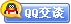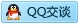用户名 Email 自动登录 找回密码 密码 会员注册
 VIP会员，3年作业免费下 ！ 奥鹏作业，奥鹏毕业论文检测 新手作业下载教程，充值问题 没有找到答案，请在此处留言！ 2019年06月最新全国统考资料 投诉建议，加盟合作！奥鹏课程积分软件(ver:3.1)

[西北工业] 西工大17春《汇编与微机接口》在线作业（资料）发表于 2017-7-26 15:38:06 | 显示全部楼层 |阅读模式西工大17春《汇编与微机接口》在线作业 一、单选题（共 37 道试题，共 92.5 分。） 1.  程序段 MOV AL，0FH XOR AL，0FFH MOV CL，7 SHR AL，CL ①上述程序段执行后，AL=（ ）H。 A. 01 B. 02 C. 03 D. 04 2.  在汇编语言程序的开发过程中使用宏功能的顺序是( ) A. 宏定义，宏调用 B. 宏定义，宏展开 C. 宏定义，宏调用，宏展开 D. 宏定义，宏展开，宏调用 3.  下列传送指令中有语法错误的是 ( ) A. MOV CS，AX B. MOV DS，AX C. MOV SS，AX D. MOV ES，AX 4.  IBM PC微机中，有符号数表示是用（ ） A. 原码 B. 补码 C. 反码 D. BCD码 5.  01100100B,不能表示的是（ ） A. 64H B. 100 C. d D. 'F 6.  已知(DS)=4000H,(SI)=1000H,COUNT=2000H,(CS)=3000H，在执行 MOV AX,[COUNT+SI] 指令时，源操作数的物理地址是 ( ) A. 43000H B. 33000H和3300H C. 43000H和43001H D. 03000H 7.  下面的数据传送指令中，错误的操作是（ ） A. MOV SS:[BX+DI],1000H B. MOV DX,1000H C. MOV WORD PTR[BX],1000H D. MOV DS,2000H 8.  汇编语言语句格式中，对名字项的规定，请指出错误的解释（ ） A. 名字的第一个字符只可以是大小写英文字母及？、＠、__等 B. 名字的第一个字符可以是大小写英文字母、数字、？、＠、__等 C. 名字的有效长度≤31个字符 D. 名字从第二个字符起可以出现数字，但不允许出现＄、#等字符 9.  在汇编语言程序中，对END语句的叙述正确的是（ ） A. END语句是一可执行语句 B. END语句表示程序执行到此结束 C. END语句表示源程序到此结束 D. END语句在汇编后要产生机器码 10.  可用于寄存器间接寻址和寄存器相对寻址的寄存器是 ( ) A. AX B. BX C. CX D. DX 11.  已知AX=0A33AH，DX=0F0F0H AND AH，DL XOR AL，DH NEG AH NOT AL 执行上述指令序列后，AH=（ ）H。 A. 60 B. 50 C. 35 D. 65 12.  有数据定义DB 4 DUP (0,2 DUP (20H))，其中能组成0020H字数据的个数是( ) A. 0 B. 3 C. 4 D. 8 13.  下面有语法错误的指令是（ ） A. MOV AX，[BX][SI] B. MOV AX，－2[BX][DI] C. MOV AX，[BX][BP] D. MOV AX，－1[BX][SI] 14.  8086存储器段最大为 （ ） A. 16K B. 32K C. 64K D. 128K 15.  已知AX=0FF60H，CF=1 MOV DX，96 XOR DH，0FFH SBB AX，DX 执行上述指令序列后，AX=（ ）。 A. 0 B. 1 C. 2 D. 3 16.  设字长为8位，补码可表示的数的范围为 （ ） A. 0--256 B. -127--+128 C. -128--+127 D. 0—128 17.  堆栈中当前出栈的存储单元的地址是（ ） A. SS×10H+SP B. SS×10H+BP C. SS×10+SP D. SS×10+BP 18.  设字长8位,数X=-10,则[X]补为 ( ) A. 0AH B. 1AH C. F5H D. F6H 19.  在AR DB 10 DUP(3,2 DUP(9))中,含数据字0309H的个数是 ( ) A. 10 B. 20 C. 0 D. 9 20.  8086/8088系统执行传送指令MOV时（ ） A. 不影响标志位 B. 影响DF方向标志 C. 影响SF符号标志 D. 影响CF进位标志 21.  程序段 XOR AL, AL DEC AL NEG AL RCL AL, 1 执行后 AL=（ ）H。 A. 01 B. 02 C. 03 D. 04 22.  汇编语言源程序中，每个语句由四项组成，如语句要完成一定功能，那么该语句中不可省略的项是( ) A. 名字项 B. 操作项 C. 操作数项 D. 注释项 23.  机器字长为8位,-127的补码是 ( ) A. 7FH B. 80H C. 81H D. OFFH 24.  已知BX=5E5CH，CX=8303H，CF=1 ROR BX，CL XOR BH，CH ROL BX，CL 上述程序段执行后，BX=（ ）H 。 A. 4658 B. 6458 C. 4568 D. 8564 25.  汇编语言语句格式中，对名字项的规定，请指出错误的解释（ ） A. 名字的第一个字符只可以是大小写英文字母及？、＠、__等 B. 名字的第一个字符可以是大小写英文字母、数字、？、＠、__等 C. 名字的有效长度≤31个字符 D. 名字从第二个字符起可以出现数字，但不允许出现＄、#等字符 26.  程序段 XOR AL,AL INC AL NEG AL MOV BL,3FH ADC AL,BL 执行后, AL=（ ）FH。 A. 1 B. 2 C. 3 D. 4 27.  设寄存器AL，BL，CL中内容均为76H XOR AL，0FH AND BL，0FH OR CL，0FH 执行上述指令序列后，AL=（ ）H。 A. 06 B. 7 C. 79 D. 97 28.  指令语句中标号的错误定义是（ ） A. 在代码段中的名字项定义，后面紧跟冒号 B. 用EQU伪指令定义 C. 用LABEL伪指令定义 D. 用“JMP 标号”指令定义 29.  语句ENDM是通知汇编程序 ( ) A. 过程定义结束 B. 宏定义结束 C. 源程序结束 D. 段定义结束 30.  MOV AL，01H ROR AL，1 OR AL，AL NEG AL 上述程序段后，CF=（ ）。 A. 1 B. 2 C. 3 D. 4 31.  下列语句中能实现留空8个字节单元的语句是（ ） A. DA1 DT ？ B. DA2 DW ？，？ C. DA3 DD ？ D. DA4 DQ ？ 32.  把汇编源程序变成目标程序的过程是（ ） A. 编译 B. 汇编 C. 编辑 D. 链接 33.  汇编语言程序中各语句的操作项可以是指令、伪指令或( ) A. 操作数 B. 运算符 C. 宏指令 D. 表达式 34.  语句ENDM是通知汇编程序为( ) A. 过程定义结束 B. 宏定义结束 C. 源程序结束 D. 段定义结束 35.  十六进制数88H，可表示成下面几种形式，请找出错误的表示（ ） A. 无符号十进制数136 B. 带符号十进制数－120 C. 压缩型BCD码十进制数88 D. 8位二进制数－8的补码表示 36.  基本ASCII码定义的字符个数为 （ ） A. 32 B. 64 C. 128 D. 256 37.  指令MOV AX, [BP][SI] ,源操作数的物理地址计算公式是( ) A. (SS)*16+(BP)+(SI) B. (DS)*16+(BP)+(SI) C. (CS)*16+(BP)+(SI) D. (ES)*16+(BP)+(SI) 西工大17春《汇编与微机接口》在线作业 二、判断题（共 3 道试题，共 7.5 分。） 1.  汇编程序能检查出程序的逻辑错误错误。 A. 错误 B. 正确 2.  对于以下宏定义 BRANCH MACRO X IF (\$-X) GE 128 JMP NEAR PTR X ELSE JMP SHORT X NDIF ENDM 现有以下代码 LOOP0: MOV AX，BX BRANCH LOOP0 宏展开LOOP0: MOV AX,BX JMP SHORT LOOP0。 A. 错误 B. 正确 3.  设有宏定义: SHIFT MACRO VAR,REG,SHF,NUM,DEST MOV REG,VAR MOV CL,NUM S&SHF REG,CL MOV DEST,REG ENDM 宏调用为: SHIFT DA_BYTE,AL,HL,CONT,DEST1; 宏扩展MOV AL, DA_BYTE MOV CL 。 A. 错误 B. 正确

本帖子中包含更多资源

x
 本版积分规则 回帖并转播 回帖后跳转到最后一页客服一客服二客服三客服四微信客服扫一扫